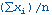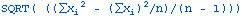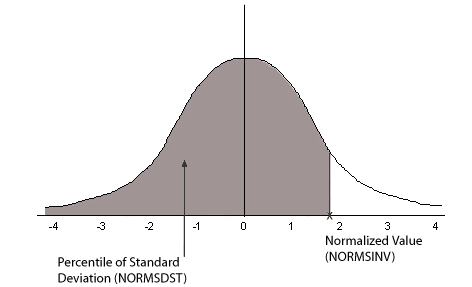NORMSDST and NORMSINV: Calculating Normal Distributions
 In this section:

The NORMSDST and NORMSINV functions perform calculations on a standard normal distribution curve. NORMSDST calculates the percentage of data values that are less than or equal to a normalized value; NORMSINV is the inverse of NORMSDST, calculates the normalized value that forms the upper boundary of a percentile in a standard normal distribution curve.

 Top of page
NORMSDST: Calculating Standard Cumulative Normal Distribution
 How to:Calculate the Cumulative Standard Normal Distribution FunctionReference: Characteristics of the Normal Distribution

The NORMSDST function performs calculations on a standard normal distribution curve, calculating the percentage of data values that are less than or equal to a normalized value. A normalized value is a point on the X-axis of a standard normal distribution curve in standard deviations from the mean. This is useful for determining percentiles in normally distributed data.

The NORMSINV function is the inverse of NORMSDST. For information about NORMSINV, see

The results of NORMSDST are returned as double-precision and are accurate to 6 significant digits.

A standard normal distribution curve is a normal distribution that has a mean of 0 and a standard deviation of 1. The total area under this curve is 1. A point on the X-axis of the standard normal distribution is called a normalized value. Assuming that your data is normally distributed, you can convert a data point to a normalized value to find the percentage of scores that are less than or equal to the raw score.

You can convert a value (raw score) from your normally distributed data to the equivalent normalized value (z-score) as follows:

`z = (raw_score - mean)/standard_deviation`

To convert from a z-score back to a raw score, use the following formula:

`raw_score = z * standard_deviation + mean`

The mean of data points xi, where i is from 1 to n is:The standard deviation of data points xi, where i is from 1 to n is:The following diagram illustrates the results of the NORMSDST and NORMSINV functions.Top of page
Reference: Characteristics of the Normal Distribution

Many common measurements are normally distributed. A plot of normally distributed data values approximates a bell-shaped curve. The two measures required to describe any normal distribution are the mean and the standard deviation:

• The mean is the point at the center of the curve.
• The standard deviation describes the spread of the curve. It is the distance from the mean to the point of inflection (where the curve changes direction).
 Top of page
Syntax: How to Calculate the Cumulative Standard Normal Distribution Function
`NORMSDST(value, 'D8');`

where:

value

Is a normalized value.

D8

Is the required format for the result. The value returned by the function is double-precision. You can assign it to a field with any valid numeric format.

 Top of page
Example: Using the NORMSDST Function

NORMSDST finds the percentile for Z and stores the result in a column with the format D8.

`NORMSDST(Z, 'D8')`

For -.07298, the result is .47091.

For -.80273 the result is .21106.

 Top of page
NORMSINV: Calculating Inverse Cumulative Normal Distribution
 How to:Calculate the Inverse Cumulative Standard Normal Distribution Function

The NORMSINV function performs calculations on a standard normal distribution curve, finding the normalized value that forms the upper boundary of a percentile in a standard normal distribution curve. This is the inverse of NORMSDST. For information about NORMSDST, see

The results of NORMSINV are returned as double-precision and are accurate to 6 significant digits.

 Top of page
Syntax: How to Calculate the Inverse Cumulative Standard Normal Distribution Function
`NORMSINV(value, 'D8');`

where:

value

Is a number between 0 and 1 (which represents a percentile in a standard normal distribution).

D8

Is the required format for the result. The value returned by the function is double-precision. You can assign it to a field with any valid numeric format.

 Top of page
Example: Using the NORMSINV Function

NORMSINV returns a normalized value from a percentile found using NORMSDST.

`NORMSINV(NORMSD, 'D8')`

For .21106, the result is -.80273.

For .47091, the result is -.07298

 iWay Software